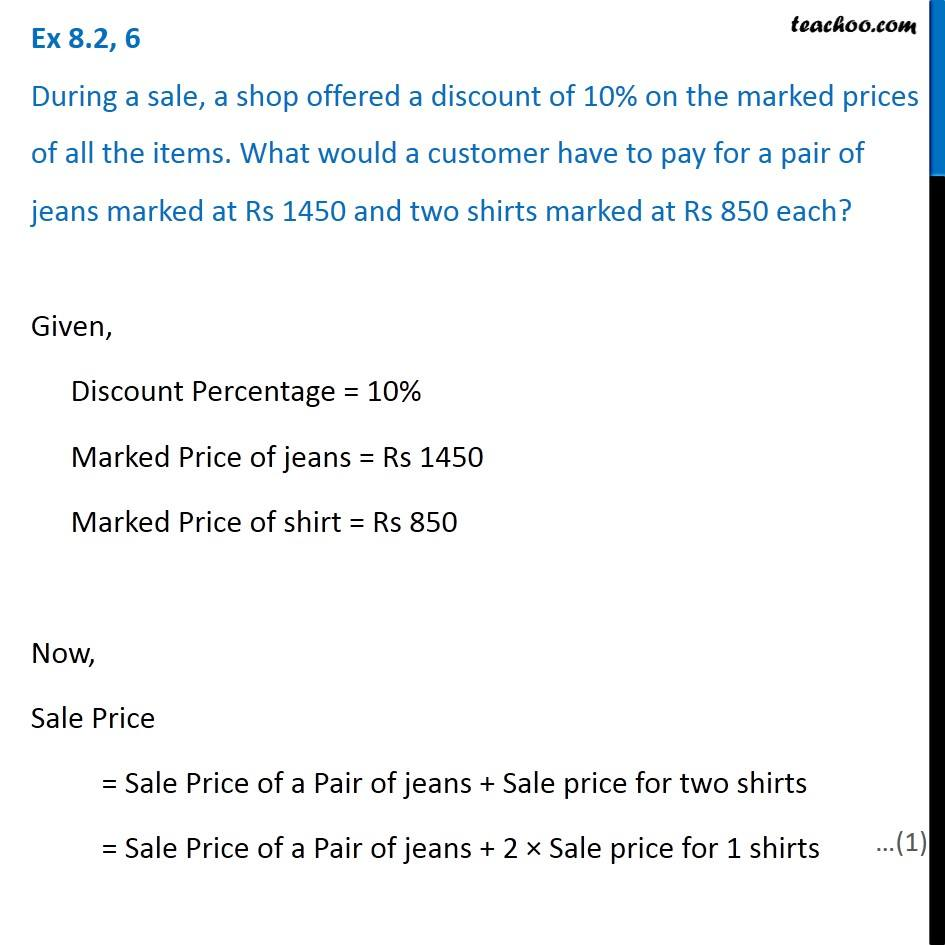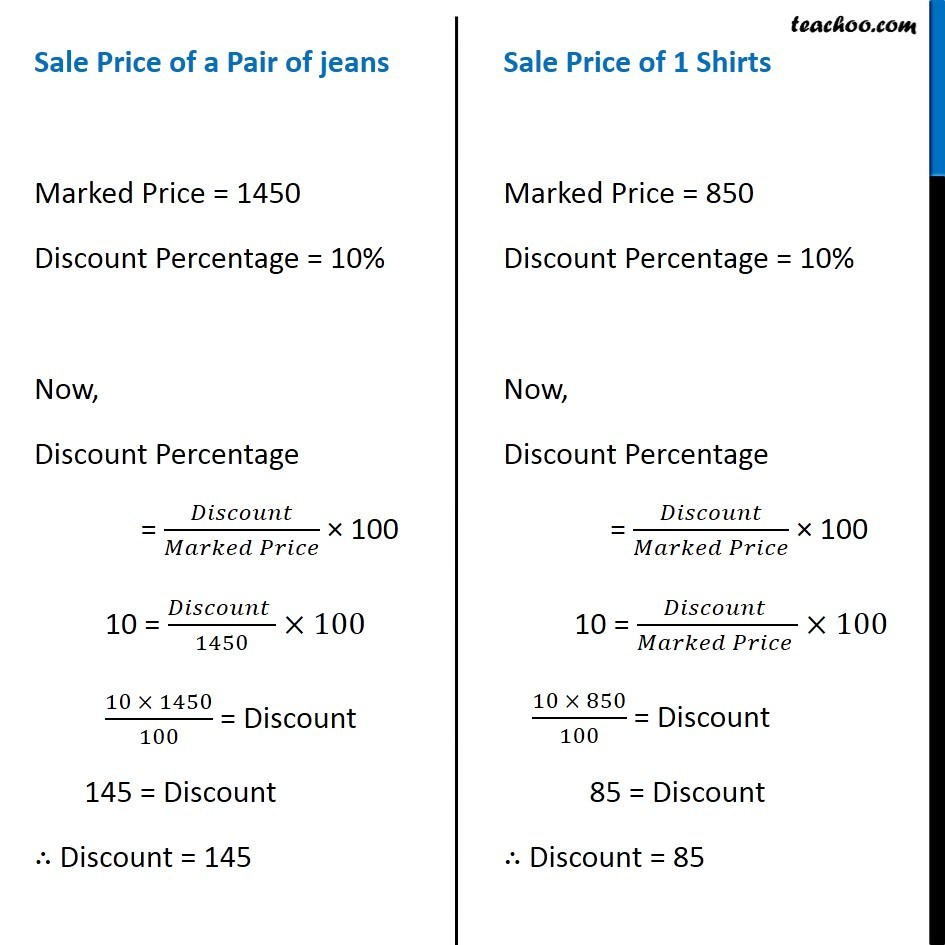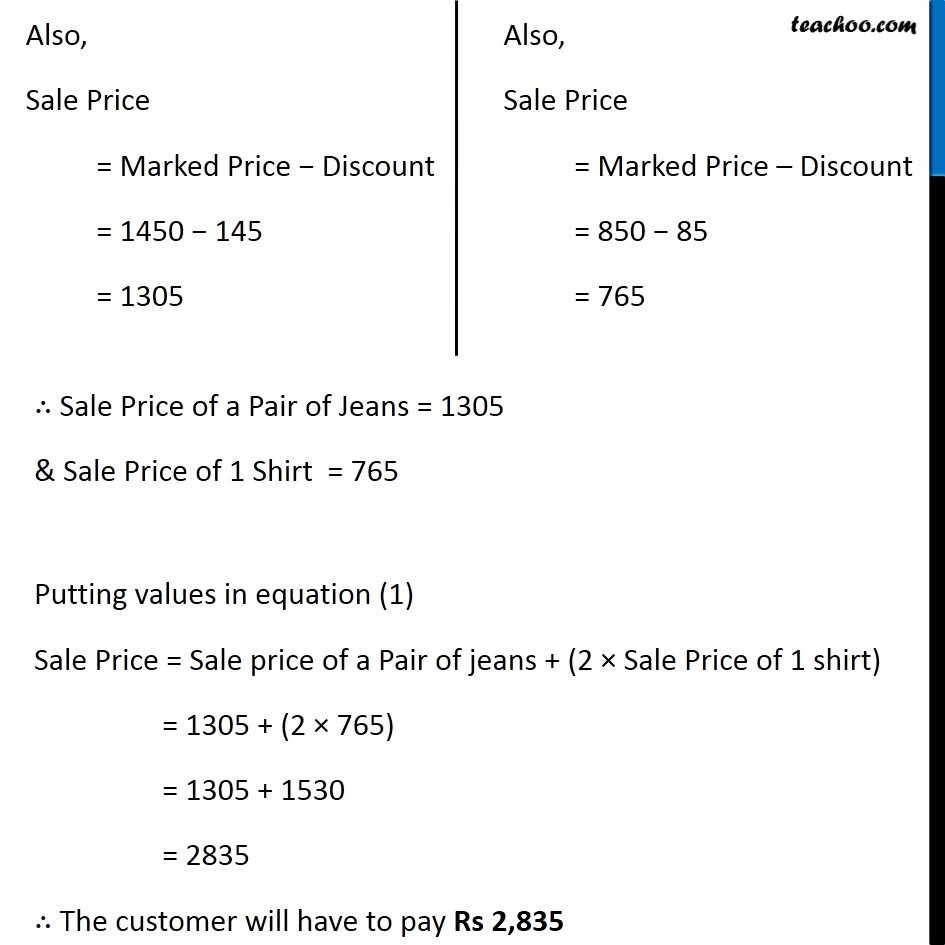1. Chapter 8 Class 8 Comparing Quantities
2. Concept wise
3. Finding Discounts

Transcript

Ex 8.2, 6 During a sale, a shop offered a discount of 10% on the marked prices of all the items. What would a customer have to pay for a pair of jeans marked at Rs 1450 and two shirts marked at Rs 850 each? Given, Discount Percentage = 10% Marked Price of jeans = Rs 1450 Marked Price of shirt = Rs 850 Now, Sale Price = Sale Price of a Pair of jeans + Sale price for two shirts = Sale Price of a Pair of jeans + 2 × Sale price for 1 shirts Sale Price of a Pair of jeans Marked Price = 1450 Discount Percentage = 10% Now, Discount Percentage = 𝐷𝑖𝑠𝑐𝑜𝑢𝑛𝑡/(𝑀𝑎𝑟𝑘𝑒𝑑 𝑃𝑟𝑖𝑐𝑒) × 100 10 = (𝐷𝑖𝑠𝑐𝑜𝑢𝑛𝑡 )/1450×100 (10 × 1450)/100 = Discount 145 = Discount ∴ Discount = 145 Sale Price of 1 Shirts Marked Price = 850 Discount Percentage = 10% Now, Discount Percentage = 𝐷𝑖𝑠𝑐𝑜𝑢𝑛𝑡/(𝑀𝑎𝑟𝑘𝑒𝑑 𝑃𝑟𝑖𝑐𝑒) × 100 10 = (𝐷𝑖𝑠𝑐𝑜𝑢𝑛𝑡 )/(𝑀𝑎𝑟𝑘𝑒𝑑 𝑃𝑟𝑖𝑐𝑒 )×100 (10 × 850)/100 = Discount 85 = Discount ∴ Discount = 85 Also, Sale Price = Marked Price − Discount = 1450 − 145 = 1305 Also, Sale Price = Marked Price – Discount = 850 − 85 = 765 ∴ Sale Price of a Pair of Jeans = 1305 & Sale Price of 1 Shirt = 765 Putting values in equation (1) Sale Price = Sale price of a Pair of jeans + (2 × Sale Price of 1 shirt) = 1305 + (2 × 765) = 1305 + 1530 = 2835 ∴ The customer will have to pay Rs 2,835

Finding Discounts